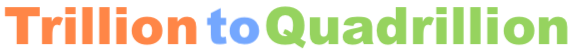Here we will convert 5.1 trillion to quadrillion. In other words, what is 5.1 trillion in terms of quadrillion? We start by showing you how to write 5.1 trillion with numbers:

5,100,000,000,000

There are one thousand trillion in a quadrillion. To give you perspective, below is how to write a quadrillion in numbers.

1,000,000,000,000,000

And here is how our 5.1 trillion fits into a quadrillion, which will illustrate how much larger a quadrillion is compared to 5.1 trillion.

1,005,100,000,000,000

Since there are one thousand trillion in a quadrillion, to convert 5.1 trillion to quadrillion, you would divide 5.1 trillion by one thousand. Here is the math and the answer:

5.1 / 1000 = 0.0051